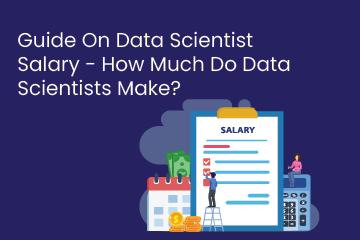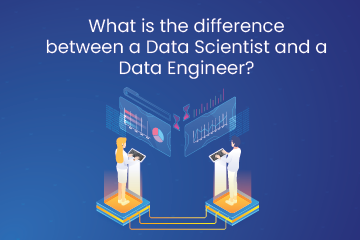Home / Blog / Data Science / A Comprehensive Guide For Data Scientists To Master The Fundamentals Of Mathematical Statistics

# A Comprehensive Guide For Data Scientists To Master The Fundamentals Of Mathematical Statistics

• February 21, 2023
• 2671
• 77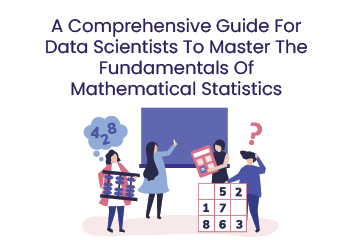### Meet the Author : Mr. Bharani Kumar

Bharani Kumar Depuru is a well known IT personality from Hyderabad. He is the Founder and Director of Innodatatics Pvt Ltd and 360DigiTMG. Bharani Kumar is an IIT and ISB alumni with more than 17 years of experience, he held prominent positions in the IT elites like HSBC, ITC Infotech, Infosys, and Deloitte. He is a prevalent IT consultant specializing in Industrial Revolution 4.0 implementation, Data Analytics practice setup, Artificial Intelligence, Big Data Analytics, Industrial IoT, Business Intelligence and Business Management. Bharani Kumar is also the chief trainer at 360DigiTMG with more than Ten years of experience and has been making the IT transition journey easy for his students. 360DigiTMG is at the forefront of delivering quality education, thereby bridging the gap between academia and industry.

Data science is a fantastic field that works with massive amounts of data using cutting-edge methods to provide helpful information. All global industries, including those in healthcare, banking, automotive, manufacturing, and education, have been completely dominated by it. According to the poll, you can expect employment in the data science field to grow significantly by 27.9 percent by 2026. For individuals with the appropriate skill set, it provides affluent career prospects with an absurdly high package and global exposure.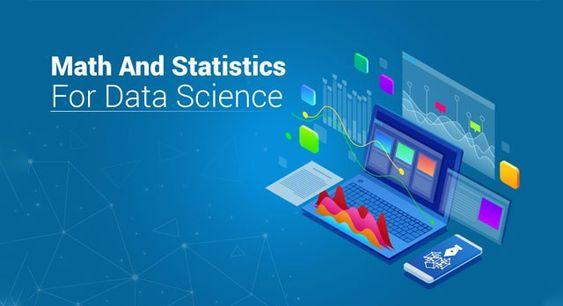Have you considered pursuing a career in data science but been put off by the necessary math skills? Even though data science is based on a lot of math, there may be less math involved than you believe in becoming a competent data scientist. It is impossible to overstate statistics' role in data science and analytics. Statistics offers tools and techniques to uncover the structure and deliver more in-depth data insights. Mathematics and statistics both like facts and detest educated guesses. When using the data to solve business challenges and create data-driven decisions, being able to think critically and creatively depends on having a firm grasp of these two crucial concepts.

Math and statistics are crucial for data science since they are the fundamental building blocks for all machine learning algorithms. Everything around us, including shapes, patterns, and colors, as well as the number of petals in flowers, is based on mathematics. Every area of our life involves mathematics.

To become a data scientist, one should compulsorily have a solid grasp of programming languages, machine learning methods, and a data-driven approach. Still, data science is about more than just these things. In this article, you will learn the value of maths and statistics for data science and how to apply them to create machine learning models.

### Introduction to Statistics:

You must have a solid foundation to succeed as a data scientist. The foundation of machine learning algorithms is math and statistics. Therefore, it is essential to be familiar with their underlying techniques to understand how and when to employ different Machine Learning algorithms—the question of what statistics now arises.

Data collection, analysis, interpretation, and presentation are all aspects of mathematical science known as statistics.

Data scientists and analysts can find significant trends and changes in data by using statistics to process complex problems in the real world and using statistics to analyze data and obtain relevant insights using mathematical computations.

Several statistical functions, principles, and algorithms are used to examine raw data, create a statistical model, and infer or forecast the outcome.

The area of statistics impacts all facets of life; just a few examples include the stock market, the life sciences, weather, retail, insurance, and education.

### Math for Data Science:

Mathematics impacts every discipline. However, the extent to which mathematics is used differs between fields. For example, linear algebra and calculus are the two main branches of mathematics that go into data science.

This section on mathematics for data science will provide a brief introduction to these two areas and explain how they benefit data research.

• ### Linear Algebra:

It is the primary subject of data science. Typical linear algebra applications include text analysis, dimensionality reduction, and image recognition. Think about a scenario of two pictures, one of a cat and the other of a dog:

Can you identify which image belongs to the cat and which to the dog? Yes, you can, without a doubt! It is because our brains are programmed to distinguish between cats and dogs from birth. As a result, we rely on intuition to conclude from the facts.

But what if you had to create a system that could distinguish between cats and dogs? The most well-known use of machine learning is for this task, which is referred to as classification. For example, the computer can distinguish between photographs of cats and dogs using linear algebra.

This image is kept there as matrices. The most crucial element of linear algebra is these matrices. One can use linear algebra to answer issues involving linear equations. Higher dimension variables may occasionally be present in these equations.

• ### Calculus:

Calculus is a crucial component of math for data science. The main application of calculus is in optimization methods.

With calculus, you can have a thorough understanding of machine learning.

You can use calculus to mathematically model artificial neural networks, improving their performance and accuracy. Calculus is categorized into –

• Differential Calculus:

Differential calculus examines the rate of change of quantities. A derivate is the most common way to get a function's maximum and minimum. One can use derivates in optimization techniques where it is necessary to discover the minima and minimize the error function.

Another key derivation concept you must comprehend is the use of partial derivations in neural network backpropagation.

Finally, another crucial idea utilized to calculate backpropagation is the chain rule.

For Generative Adversarial Neural Networks, we apply differential game theory in addition to backpropagation and minimizing error functions.

• Integral Calculus:

The mathematical study of the accumulation of quantities and for calculating the area under the curve is known as integral calculus. Different types of integrals include definite and indefinite integrals.

The most common applications of integration are in the computation of the variance and probability density functions of random variables. However, Bayesian inference is another significant application of integral calculus in machine learning.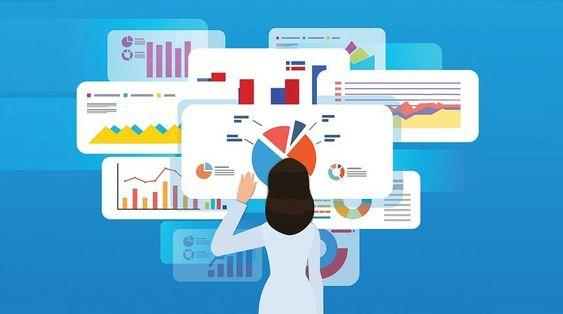### Statistics for Data Science:

The study of data gathering, analysis, visualization, and interpretation is known as statistics. A powerful sports car that operates on statistics is what data science is like. Using statistics, it turns raw data into the insights that make up the data products.

Statistics deals with unprocessed data and aids businesses in making thoughtful data-driven decisions. Numerous tools and capabilities offered by statistics can assist you in locating a vast amount of data.

Additionally, you can gain a profound understanding of the data by using statistics for data summarization and inference. In terms of these two terms, statistics are split into two categories –

• Descriptive Statistics
• Inferential Statistics
• Descriptive Statistics:

One can use descriptive statistics or summary statistics to describe the data. It deals with analyzing data quantitatively and summarizing it. To summarize, you can use graphs or numerical representations.

• Inferential Statistics:

Inferential statistics can be referred the process of drawing conclusions or inferences from data. For example, we conduct numerous tests and draw inferences from the smaller sample using inferential statistics to conclude the broader population.

For instance, you should know how many individuals favor a particular political party during an election survey. But, of course, you need to ask everyone their opinions to do this.

This method is incorrect because billions of individuals live in India, making it impossible to poll every one of them. As a result, we choose a smaller sample, draw conclusions from it, and then apply those conclusions to the entire population to explain our results.

### Mathematical Concepts You Should Understand for Data Science & Machine Learning

• Basic algebra: linear, exponential, logarithmic, and other functions; variables; coefficients; equations; and so forth.
• Linear algebra: scalars, vectors, tensors, Norms (L1 & L2), dot product, types of matrices, linear transformation, expressing linear equations in matrix notation, and solving linear regression problems with vectors and matrices.
• Calculus: limits and derivatives, derivative rules, the chain rule (for the backpropagation process), partial derivatives (to compute gradients), convexity of functions, local/global minima, the mathematics behind a regression model, and applied math for building a model from scratch.

### Essential Statistics for Machine Learning and Data Science

Today, every organization aspires to be data-driven. Data scientists and analysts must use their data to inform their decision-making in various ways.

• From data to insights: A description of data: Data always arrives in its raw, unsightly form. The initial investigation reveals what's missing, how you can disperse the data and the best method for cleaning it to get the desired outcome. Descriptive statistics allow you to interpret each observation in your data to provide answers to the specified queries.
• How to measure uncertainty: Additionally, you must be able to measure uncertainty. Any data organization would value this ability highly because it is so important. Understanding the likelihood of any experiment or choice succeeding is crucial for all firms.

### Statistics terminology - Statistics for Data Science:

When working with Statistics for Data Science, it is essential to understand a few basic statistical terminologies. Below, I've explained these terms:

• The population is the group of sources from whom one must gather the information.
• A sample is referring to a subset of the population.
• Anything that can be measured or quantified as a characteristic, number, or amount is considered a variable.
• A statistical model is another name for A variable that indexes a family of probability distributions known as a statistical parameter or population parameter. For instance, a population's mean, median, etc.### Data Representation in Statistics:

Data is the term for a group of observations and facts. One may express these observations and facts as measurements, assertions, or numerical data.

Data can be split into two categories: qualitative data and quantitative data. Quantitative data is numerical information, while qualitative data is descriptive or categorical information. Once we know the techniques used to obtain the data, we aim to visualize the data in various graphs, including bar graphs, line graphs, pie charts, stem, leaf plots, scatter plots, and more. You can eliminate the outliers before analysis resulting from the variability in the data measurements.

### Various Models of Statistics:

Since the term "statistics" is used in many different contexts, various statistical models are employed. Here are some examples of models:

Skewness - In statistics, the term "skewness" refers to a measure of the asymmetry in a probability distribution where it quantifies the deviation of the normal distribution curve for data. Skewed distribution values might be positive, negative, or zero. When the curve shifts from left to right, it is considered skewed. Positive skewness refers to a curve moving more to the right, whereas left skewness refers to a curve moving more to the left.

ANOVA Statistics - The term ANOVA stands for Analysis of Variance. The ANOVA statistic is the unit of analysis used to determine the mean difference for the specified data set. One can compare the performance of stocks over time using this statistical approach.

Degrees of freedom: This statistical model is applied when the values. The degree of freedom is information that one can change during parameter estimation.

Regression Analysis: The statistical procedure establishes the correlation between the variables in this model. The process illustrates how a dependent variable changes due to an altered independent variable.### Pursue Your Mathematical Statistics and Data Science Education through 360digiTMG:

Because machine learning algorithms, data analysis, and finding insights from data all involve math, professions in data science necessitate mathematical studies. While not the only prerequisite for your degree and future in data science, math is sometimes one of the most crucial. For example, it is generally agreed that one of the most crucial tasks in a data scientist's workflow is recognizing, comprehending, and translating business difficulties into quantitative ones.

No matter what business you plan to work in after graduation, math is a fundamental educational requirement for data scientists. It guarantees you can efficiently apply complicated data to address business problems, assist an organization in innovating more quickly, and improve model performance.

Use a reputable online course provider like 360digiTMG to ensure you're developing the appropriate skill sets and mathematical capabilities. They provide mathematics and data science certification courses that will walk you through all you need to know to pursue a career in data science.

### Data Analyst Courses in Other Locations